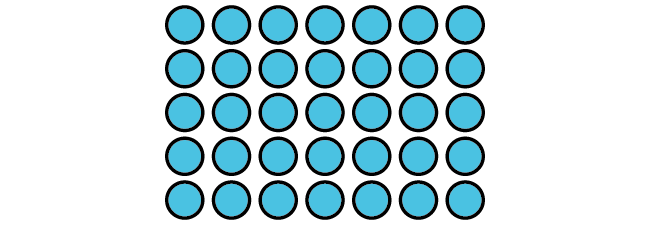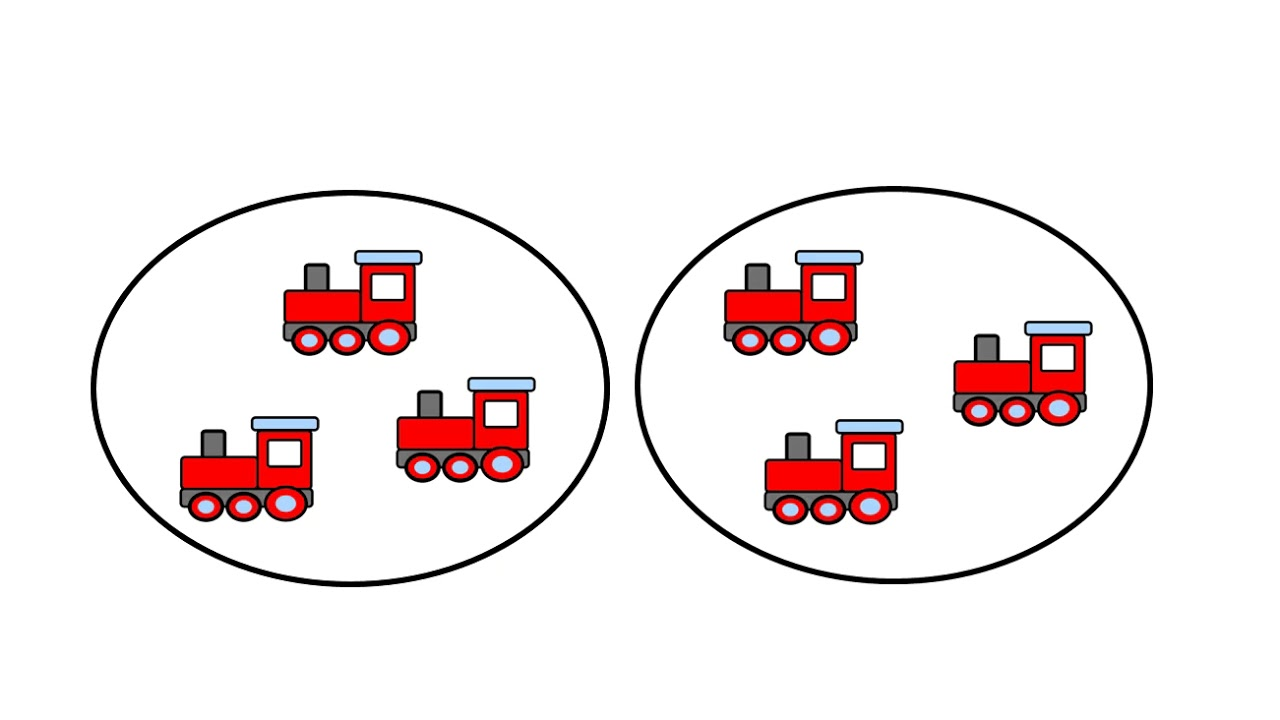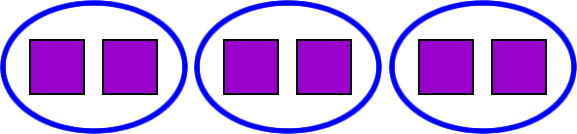Arrays
Multiplication
Division
Equal Groups
100

Write a multiplication equation for this array:5 x 7 = 35

100

Solve the problem:

3x9=

27
100

Solve this problem:

164

4

100

Write a multiplication equation for this problem:

4 + 4 + 4

3 x 4 = 12

100

Write a multiplication equation for these equal groups:2 x 3 = 6

200

Write a multiplication equation for this array:5 x 3 = 15

200

Solve the problem:

5x8=

40

200

Solve this problem:

217

3

200

Write a multiplication equation for this problem:

3 + 3 + 3 + 3 + 3 + 3 + 3

7 x 3 = 21

200

Write a multiplication equation for these equal groups:4 x 5 = 20

300

Write a multiplication equation for this array:2 x 6 = 12

300

Is this problem correct

3x6=36

No the correct answer is 18

300

Solve this problem:

142

7

300

Write a multiplication equation for this problem:

6 + 6

2 x 6 = 12

300

Write a multiplication equation for these equal groups:3 x 2 = 6

400

Write a multiplication equation for each of these arrays:3 x 5 = 15   and   5 x 3 = 15

400

Solve the problem:

9x7=

63

400

Solve the problem:

153

5

400

Write a multiplication equation for this problem:

10 + 10 + 10 + 10

4 x 10 = 40

400

Write a multiplication equation for these groups:3 x 6 = 18

500

Solve the problem:

8x 9=

72

500

Is this problem correct

10x10=10

No the correct answer is 100

500

Solve the problem:

9010

9

500

Write a multiplication equation for this problem:

1 + 2 + 3 + 4 + 5

You can't because they're not the same numbers!

500

Write a multiplication equation for these groups:You can't because they're not equal groups!

Click to zoom